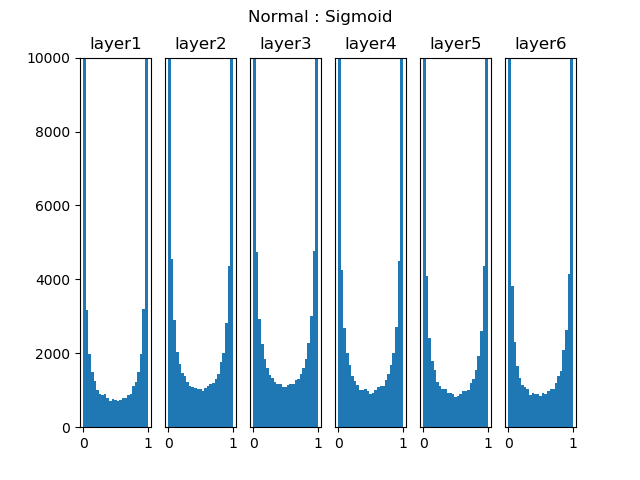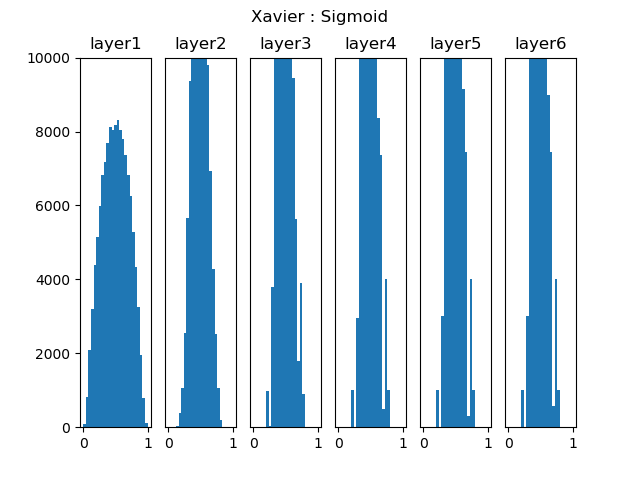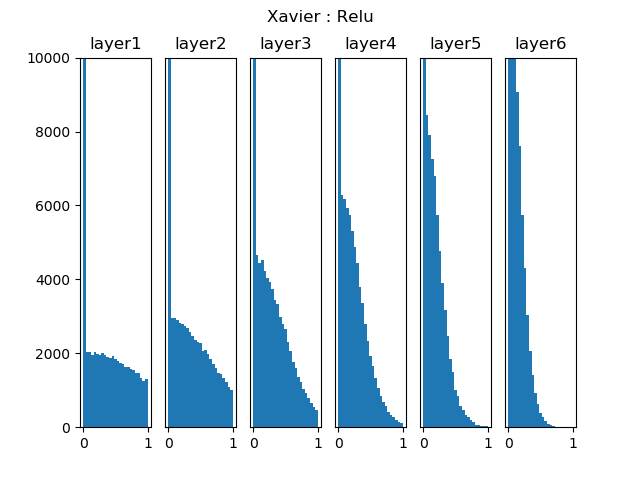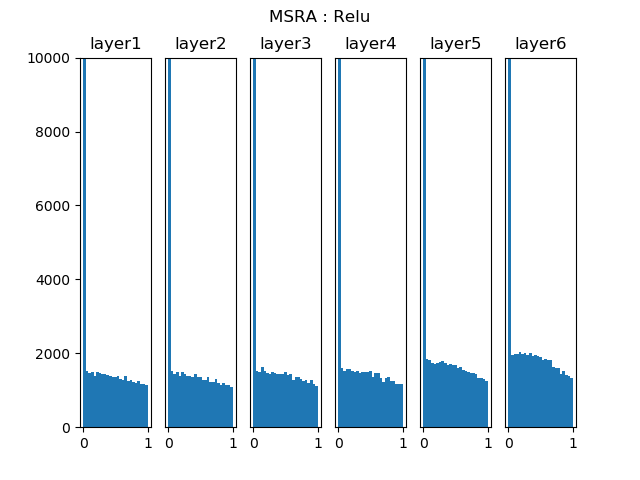# #优质创作者# 深度学习基础-网络层参数初始化详解 原创

1收藏

【本文正在参加优质创作者激励计划】

## 二，权重初始化方式分类

1. 加载预训练模型参数初始化：直接加载在大规模数据集上训练得到模型参数，一定程度上提升模型的泛化能力。
2. 随机初始化：注意不能将参数值全部初始化为 0，因为如果神经网络第一遍前向传播所有隐层神经网络激活值相同，反向传播权重更新也相同，导致隐藏层的各个神经元没有区分性，导致“对称权重”现象。较好的方式是对每个参数进行随机初始化
3. 固定参数值初始化：比如对于偏置（bias）通常用 0 初始化，LSTM 遗忘门偏置通常为 1或 2，使时序上的梯度变大，对于 ReLU 神经元，偏置设为 0.01，使得训练初期更容易激活。

### 2.1，全零初始化

W1= [[-0.82452497 -0.82452497 -0.82452497]]
B1= [[-0.01143752 -0.01143752 -0.01143752]]
W2= [[-0.68583865]
[-0.68583865]
[-0.68583865]]
B2= [[0.68359678]] # 单个输出的的双层神经网络


### 2.2，标准初始化

1，高斯分布初始化：使用高斯分布对每个参数随机初始化，即将权重 $W$ 按如下公式进行初始化:

$$W \sim N[0, \sigma^2]$$

Pytorch 框架中对应的 API 如下。

# 一般默认采用标准高斯分布初始化方法，即均值为 0，方差为 1，
torch.nn.init.normal_(tensor, mean=0, std=1)


2，与高斯分布初始化方式类似的是均匀分布初始化，其参数范围区是 $[-r, r]$。

Pytorch 框架中对应的 API 如下。

torch.nn.init.uniform_(tensor, a=0, b=1)


• 如果太小，会导致神经元输出过小，经过多层则梯度信号消失了。
• 如果太大，sigmoid 激活梯度接近 0，导致梯度消失。一般需要配合 BN 层一起使用。### 2.3，Xavier 初始化

Xavier 初始化方法比直接用高斯分布进行初始化 $W$ 的优势在于：一般的神经网络在前向传播时神经元输出值的方差会不断增大，而使用 Xavier 等方法理论上可以保证每层神经元输入输出数据分布方差一致（和 BN 层效果类似，具体原理参考Xavier 论文）。

$$W \sim N \begin{pmatrix} 0, \sqrt{\frac{2}{n_{in} + n_{out}}} \end{pmatrix}$$

Pytorch 框架中对应的 API 如下。

torch.nn.init.xavier_normal_(tensor, gain=1)


$$W \sim U \begin{pmatrix} -\sqrt{\frac{6}{n_{in} + n_{out}}}, \sqrt{\frac{6}{n_{in} + n_{out}}} \end{pmatrix}$$### 2.4，He 初始化He 初始化法最主要是想解决使用 ReLU 激活函数后，方差会发生变化的问题。

$$W \sim N \begin{pmatrix} 0, \sqrt{\frac{2}{n}} \end{pmatrix}$$

Pytorch 框架中对应的 API 如下。

torch.nn.init.kaiming_normal_(tensor, a=0, mode='fan_in', nonlinearity='leaky_relu')### 2.5，总结

• Xavier 初始化和 He 初始化目的都是想可以根据神经元连接数量自适应调整初始化分布的方差，也称为“方差缩放”
• 网络参数初始化方法的选择对保持数值稳定性至关重要，其与非线性激活函数的选择得结合一起考虑。
• 网络参数初始化的选择可以决定优化算法收敛的速度有多快。糟糕选择可能会导致我们在训练神经网络模型时遇到梯度爆炸或梯度消失。

## 参考资料